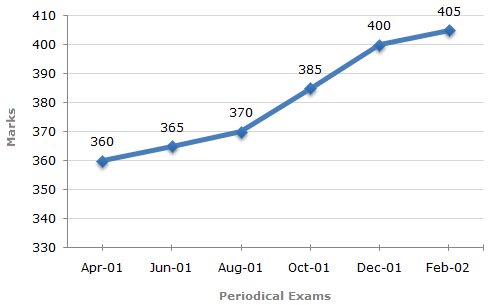# Data Interpretation - Line Charts

Exercise : Line Charts - Line Chart 10
Directions to Solve

In a school the periodical examination are held every second month. In a session during April 2001 - March 2002, a student of Class IX appeared for each of the periodical exams. The aggregate marks obtained by him in each perodical exam are represented in the line-graph given below.

Marks Obtained by student in Six Periodical Held in Every Two Months During the Year in the Session 2001 - 2002.

Maximum Total Marks in each Periodical Exam = 5001.
In which periodical exams did the student obtain the highest percentage increase in marks over the previous periodical exams ?
June, 01
August, 01
Oct, 01
Dec, 01
Explanation:

Percentage increase in marks in various periodical exams compared to the previous exams are:

 For Jun 01 =(365 - 360) x 100% = 1.39%. 360

 For Aug 01 =(370 - 365) x 100% = 1.37%. 365

 For Oct 01 =(385 - 370) x 100% = 4.05%. 370

 For Dec 01 =(400 - 385) x 100% = 3.90%. 385

 For Feb 02 =(404 - 400) x 100% = 1.25%. 400

Clearly, highest percentage increase in marks is in Oct 01.

2.
The total number of marks obtained in Feb. 02 is what percent of the total marks obtained in April 01 ?
110%
112.5%
115%
116.5%
Explanation:

Here it is clear from the graph that the student obtained 360, 365, 370, 385, 400 and 405 marks in periodical exams held in Apr 01, Jun 01, Aug 01, Oct 01, Dec 01 and Feb 02 respectively.

 Required percentage =405 x 100% = 112.5%. 360

3.
What is the percentage of marks obtained by the student in the periodical exams of August, 01 and Oct, 01 taken together ?
73.25%
75.5%
77%
78.75%
Explanation:

 Required percentage =(370 + 385) x 100% =755 x 100% = 75.5%. (500 + 500) 1000

4.
What are the average marks obtained by the student in all the periodical exams during the last session ?
373
379
381
385
Explanation:

Average marks obtained in all the periodical exams

 = 1 x [360 + 365 + 370 + 385 + 400 + 405] = 380.83381. 6

5.
In which periodical exams there is a fall in percentage of marks as compared to the previous periodical exams ?
None
June, 01
Oct, 01
Feb, 02